# Extract and Visualize the Results of Multivariate Data Analyses

Provides some easy-to-use functions to extract and visualize the output of multivariate data analyses, including 'PCA' (Principal Component Analysis), 'CA' (Correspondence Analysis), 'MCA' (Multiple Correspondence Analysis), 'FAMD' (Factor Analysis of Mixed Data), 'MFA' (Multiple Factor Analysis) and 'HMFA' (Hierarchical Multiple Factor Analysis) functions from different R packages. It contains also functions for simplifying some clustering analysis steps and provides 'ggplot2' - based elegant data visualization.

factoextra is an R package making easy to extract and visualize the output of exploratory multivariate data analyses, including:

1. Principal Component Analysis (PCA), which is used to summarize the information contained in a continuous (i.e, quantitative) multivariate data by reducing the dimensionality of the data without loosing important information.

2. Correspondence Analysis (CA), which is an extension of the principal component analysis suited to analyse a large contingency table formed by two qualitative variables (or categorical data).

3. Multiple Correspondence Analysis (MCA), which is an adaptation of CA to a data table containing more than two categorical variables.

4. Multiple Factor Analysis (MFA) dedicated to datasets where variables are organized into groups (qualitative and/or quantitative variables).

5. Hierarchical Multiple Factor Analysis (HMFA): An extension of MFA in a situation where the data are organized into a hierarchical structure.

6. Factor Analysis of Mixed Data (FAMD), a particular case of the MFA, dedicated to analyze a data set containing both quantitative and qualitative variables.

There are a number of R packages implementing principal component methods. These packages include: FactoMineR, ade4, stats, ca, MASS and ExPosition.

However, the result is presented differently according to the used packages. To help in the interpretation and in the visualization of multivariate analysis - such as cluster analysis and dimensionality reduction analysis - we developed an easy-to-use R package named factoextra.

• The R package factoextra has flexible and easy-to-use methods to extract quickly, in a human readable standard data format, the analysis results from the different packages mentioned above.
• It produces a ggplot2-based elegant data visualization with less typing.
• It contains also many functions facilitating clustering analysis and visualization.

The figure below shows methods, which outputs can be visualized using the factoextra package. The official online documentation is available at: http://www.sthda.com/english/rpkgs/factoextra.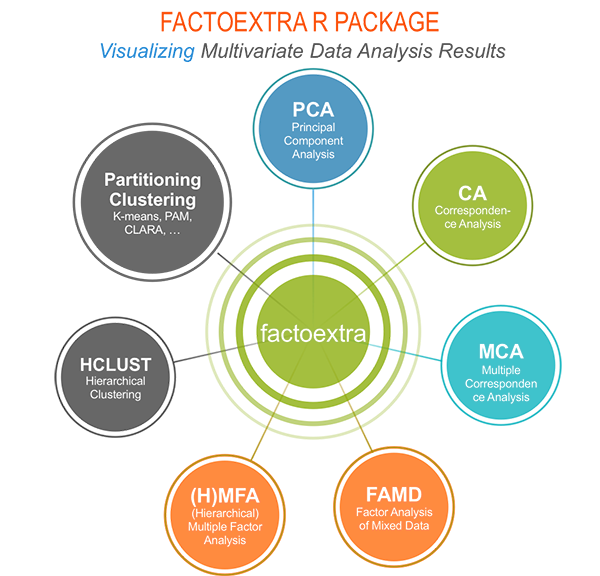## Why using factoextra?

1. The factoextra R package can handle the results of PCA, CA, MCA, MFA, FAMD and HMFA from several packages, for extracting and visualizing the most important information contained in your data.

2. After PCA, CA, MCA, MFA, FAMD and HMFA, the most important row/column elements can be highlighted using :

• their cos2 values corresponding to their quality of representation on the factor map
• their contributions to the definition of the principal dimensions.

If you want to do this, the factoextra package provides a convenient solution.

1. PCA and (M)CA are used sometimes for prediction problems : one can predict the coordinates of new supplementary variables (quantitative and qualitative) and supplementary individuals using the information provided by the previously performed PCA or (M)CA. This can be done easily using the FactoMineR package.

If you want to make predictions with PCA/MCA and to visualize the position of the supplementary variables/individuals on the factor map using ggplot2: then factoextra can help you. It's quick, write less and do more...

1. Several functions from different packages - FactoMineR, ade4, ExPosition, stats - are available in R for performing PCA, CA or MCA. However, The components of the output vary from package to package.

No matter the package you decided to use, factoextra can give you a human understandable output.

## Installing FactoMineR

The FactoMineR package can be installed and loaded as follow:

• factoextra can be installed from CRAN as follow:

## Main functions in the factoextra package

See the online documentation (http://www.sthda.com/english/rpkgs/factoextra) for a complete list.

### Visualizing dimension reduction analysis outputs

Functions Description
fviz_eig (or fviz_eigenvalue) Extract and visualize the eigenvalues/variances of dimensions.
fviz_pca Graph of individuals/variables from the output of Principal Component Analysis (PCA).
fviz_ca Graph of column/row variables from the output of Correspondence Analysis (CA).
fviz_mca Graph of individuals/variables from the output of Multiple Correspondence Analysis (MCA).
fviz_mfa Graph of individuals/variables from the output of Multiple Factor Analysis (MFA).
fviz_famd Graph of individuals/variables from the output of Factor Analysis of Mixed Data (FAMD).
fviz_hmfa Graph of individuals/variables from the output of Hierarchical Multiple Factor Analysis (HMFA).
fviz_ellipses Draw confidence ellipses around the categories.
fviz_cos2 Visualize the quality of representation of the row/column variable from the results of PCA, CA, MCA functions.
fviz_contrib Visualize the contributions of row/column elements from the results of PCA, CA, MCA functions.

### Extracting data from dimension reduction analysis outputs

Functions Description
get_eigenvalue Extract and visualize the eigenvalues/variances of dimensions.
get_pca Extract all the results (coordinates, squared cosine, contributions) for the active individuals/variables from Principal Component Analysis (PCA) outputs.
get_ca Extract all the results (coordinates, squared cosine, contributions) for the active column/row variables from Correspondence Analysis outputs.
get_mca Extract results from Multiple Correspondence Analysis outputs.
get_mfa Extract results from Multiple Factor Analysis outputs.
get_famd Extract results from Factor Analysis of Mixed Data outputs.
get_hmfa Extract results from Hierarchical Multiple Factor Analysis outputs.
facto_summarize Subset and summarize the output of factor analyses.

### Clustering analysis and visualization

Functions Description
dist(fviz_dist, get_dist) Enhanced Distance Matrix Computation and Visualization.
get_clust_tendency Assessing Clustering Tendency.
fviz_nbclust(fviz_gap_stat) Determining and Visualizing the Optimal Number of Clusters.
fviz_dend Enhanced Visualization of Dendrogram
fviz_cluster Visualize Clustering Results
fviz_mclust Visualize Model-based Clustering Results
fviz_silhouette Visualize Silhouette Information from Clustering.
hcut Computes Hierarchical Clustering and Cut the Tree
hkmeans (hkmeans_tree, print.hkmeans) Hierarchical k-means clustering.
eclust Visual enhancement of clustering analysis

## Dimension reduction and factoextra

As depicted in the figure below, the type of analysis to be performed depends on the data set formats and structures.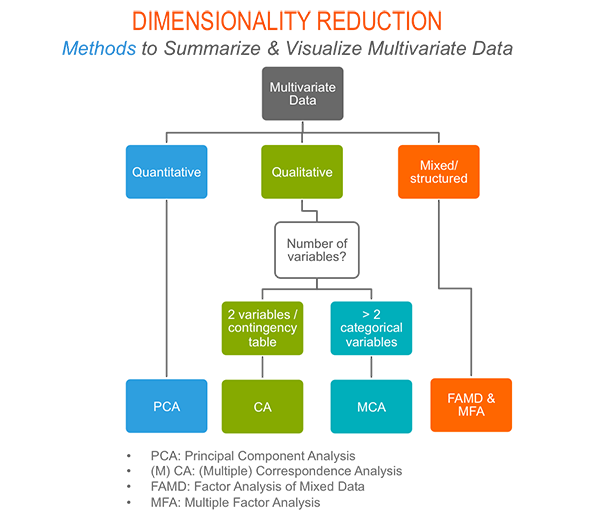In this section we start by illustrating classical methods - such as PCA, CA and MCA - for analyzing a data set containing continuous variables, contingency table and qualitative variables, respectively.

We continue by discussing advanced methods - such as FAMD, MFA and HMFA - for analyzing a data set containing a mix of variables (qualitatives & quantitatives) organized or not into groups.

Finally, we show how to perform hierarchical clustering on principal components (HCPC), which useful for performing clustering with a data set containing only qualitative variables or with a mixed data of qualitative and quantitative variables.

### Principal component analysis

• Data: decathlon2 [in factoextra package]
• PCA function: FactoMineR::PCA()
• Visualization factoextra::fviz_pca()

Read more about computing and interpreting principal component analysis at: Principal Component Analysis (PCA).

1. Principal component analysis
1. Extract and visualize eigenvalues/variances: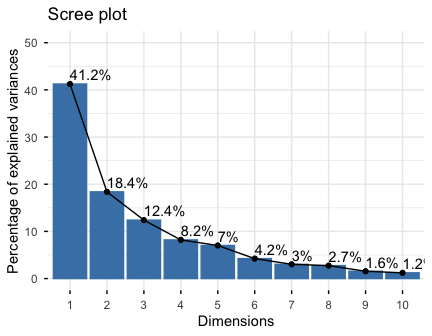4.Extract and visualize results for variables: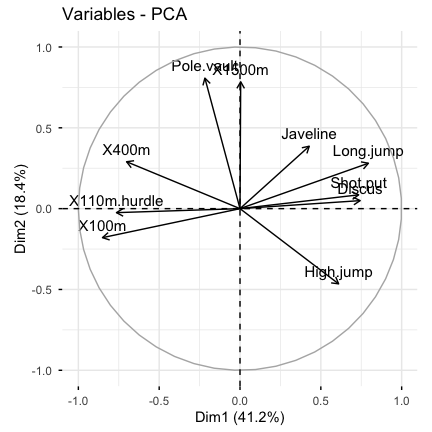It's possible to control variable colors using their contributions ("contrib") to the principal axes: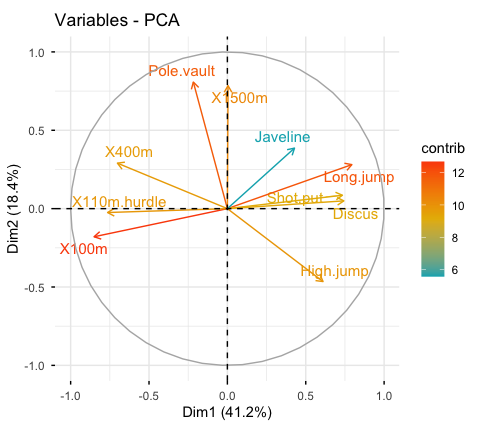1. Variable contributions to the principal axes: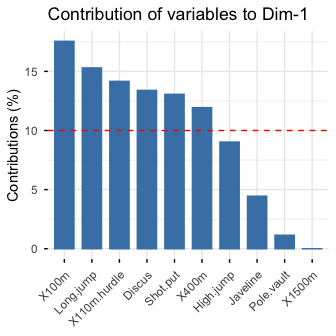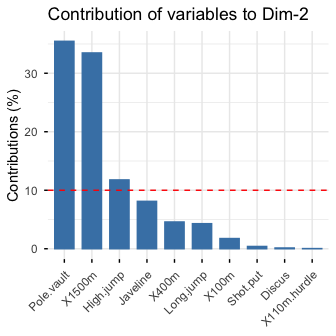1. Extract and visualize results for individuals: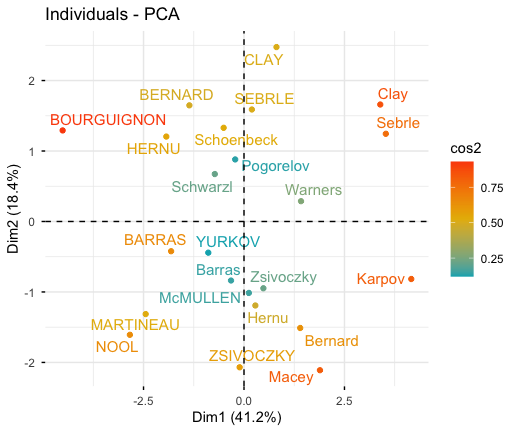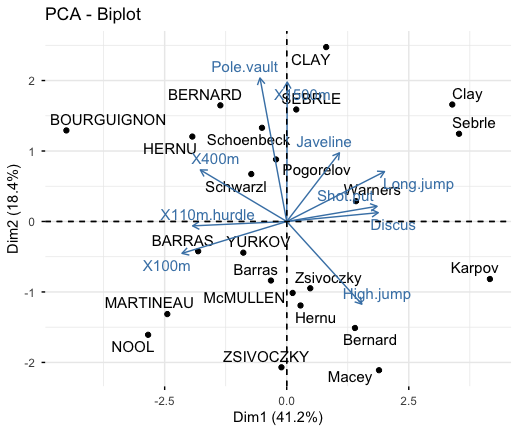1. Color individuals by groups: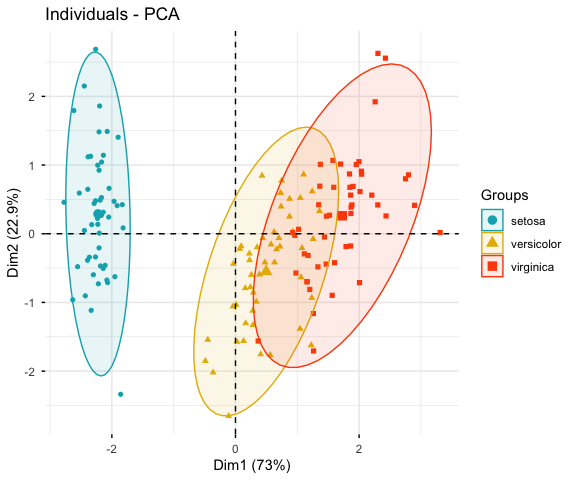### Correspondence analysis

• CA function FactoMineR::CA()
• Visualize with factoextra::fviz_ca()

Read more about computing and interpreting correspondence analysis at: Correspondence Analysis (CA).

• Compute CA:
• Extract results for row/column variables:
• Biplot of rows and columns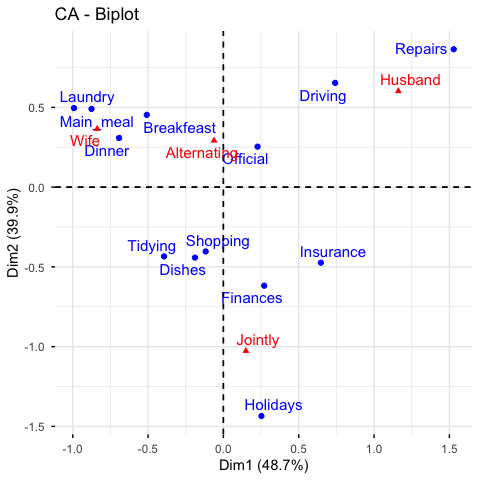To visualize only row points or column points, type this:

### Multiple correspondence analysis

• Data: poison [in factoextra]
• MCA function FactoMineR::MCA()
• Visualization factoextra::fviz_mca()

Read more about computing and interpreting multiple correspondence analysis at: Multiple Correspondence Analysis (MCA).

1. Computing MCA:
1. Extract results for variables and individuals:
1. Contribution of variables and individuals to the principal axes:
1. Graph of individuals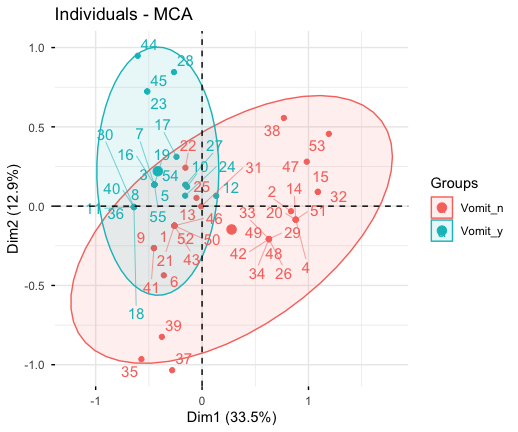1. Graph of variable categories: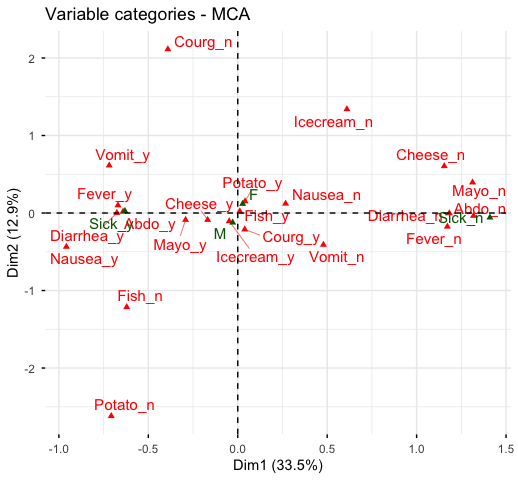1. Biplot of individuals and variables: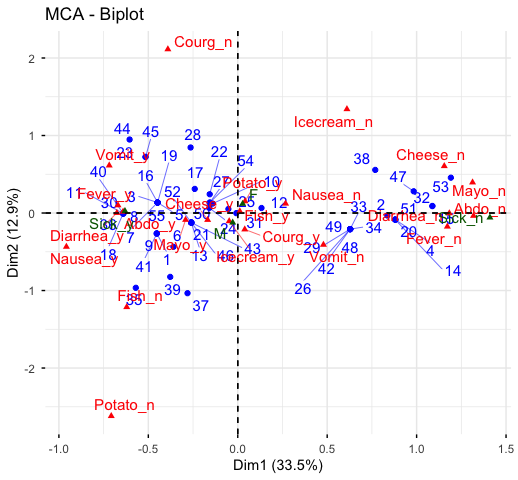The factoextra R package has also functions that support the visualization of advanced methods such:

## Cluster analysis and factoextra

To learn more about cluster analysis, you can refer to the book available at: Practical Guide to Cluster Analysis in R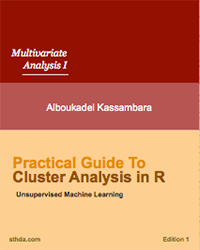The main parts of the book include:

• distance measures,
• partitioning clustering,
• hierarchical clustering,
• cluster validation methods, as well as,
• advanced clustering methods such as fuzzy clustering, density-based clustering and model-based clustering.

The book presents the basic principles of these tasks and provide many examples in R. It offers solid guidance in data mining for students and researchers.

### Partitioning clustering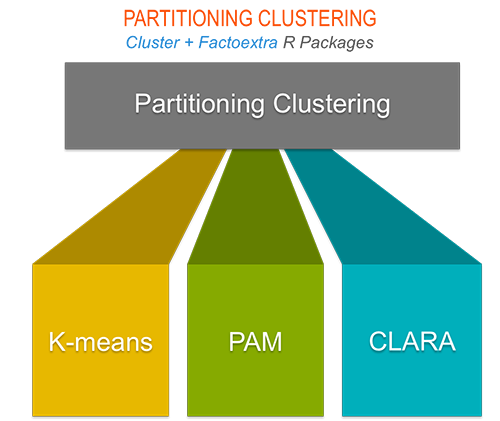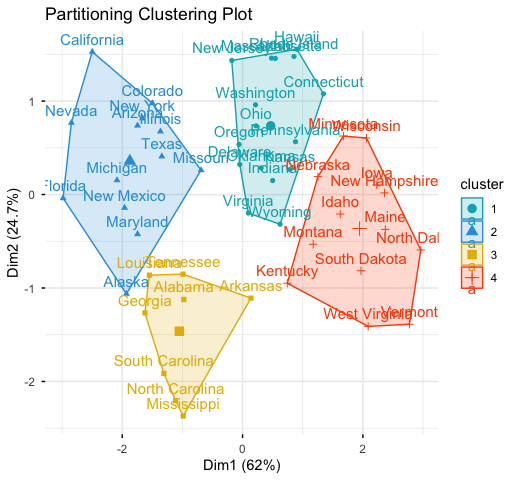### Hierarchical clustering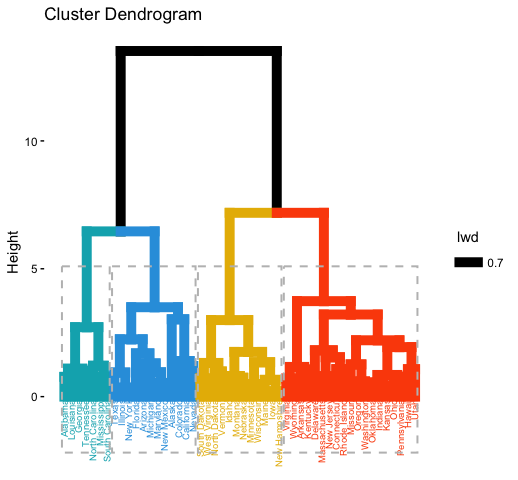### Determine the optimal number of clusters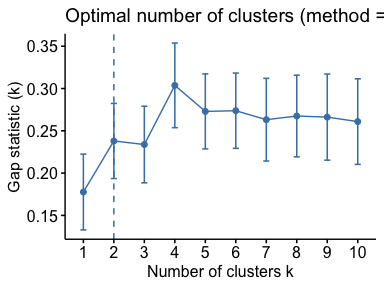## Acknoweledgment

I would like to thank Fabian Mundt for his active contributions to factoextra.

We sincerely thank all developers for their efforts behind the packages that factoextra depends on, namely, ggplot2 (Hadley Wickham, Springer-Verlag New York, 2009), FactoMineR (Sebastien Le et al., Journal of Statistical Software, 2008), dendextend (Tal Galili, Bioinformatics, 2015), cluster (Martin Maechler et al., 2016) and more .....

## References

• H. Wickham (2009). ggplot2: Elegant Graphics for Data Analysis. Springer-Verlag New York.
• Maechler, M., Rousseeuw, P., Struyf, A., Hubert, M., Hornik, K.(2016). cluster: Cluster Analysis Basics and Extensions. R package version 2.0.5.
• Sebastien Le, Julie Josse, Francois Husson (2008). FactoMineR: An R Package for Multivariate Analysis. Journal of Statistical Software, 25(1), 1-18. 10.18637/jss.v025.i01
• Tal Galili (2015). dendextend: an R package for visualizing, adjusting, and comparing trees of hierarchical clustering. Bioinformatics. DOI: 10.1093/bioinformatics/btv428

# factoextra 1.0.5

## Bug fixes

• Now, the argument `invisible` works properly in the function `fviz_pca_biplot()`(@ginolhac, #26).
• The function `fviz_dend()` now works for an object of class `diana` (@qfazille, #30).
• Now, `fviz_cluster()` supports HCPC results (@famuvie, #34).

## Minor changes

• New argument `mean.point` in the function `fviz()`. logical value. If TRUE, group mean points are added to the plot.

• Now, PCA correlation circles have fixed coordinates so they don't appear as ellipses (@scoavoux, #38.

• New argument `fill.ind` and `fill.var` added in `fviz_pca()` (@ginolhac, #27 and @Confurious, #42).

• New arguments `geom.ind` and `geom.var` in `fviz_pca_xxx()` and `fviz_mca_xxx()` functions to have more controls on the individuals/variables geometry in the functions `fviz_pca_biplot()` and `fviz_mca_biplot()` (@Confurious, #42).

• New arguments `geom.row` and `geom.col` in `fviz_ca_xxx()` functions to have more controls on the individuals/variables geometry in the function `fviz_ca_biplot()` (@Confurious, #42).

• New argument `gradient.cols` in `fviz_pca_biplot()`

• New argument `àxes` in `fviz_cluster`() to specify the dimension to plot.

• New argument `circlesize` in the function `fviz()` to change the size of the variable correlation circle size.

• It's now possible to color individuals using a custom continuous variable (#29). This is done using the argument col.ind.

• factoextra can now handle Japanese characters by using the argument font.family = "HiraKakuProN-W3"` (#31). For example:

# factoextra 1.0.4

## New features

• New function `fviz_mclust()` for plotting model-based clustering using ggplot2.

• New function `fviz()`: Generic function to create a scatter plot of multivariate analyse outputs, including PCA, CA and MCA, MFA, ...

• New functions `fviz_mfa_var()` and `fviz_hmfa_var()` for plotting MFA and HMFA variables, respectively.

• New function `get_mfa_var()`: Extract the results for variables (quantitatives, qualitatives and groups). Deprecated functions: `get_mfa_var_quanti()`, `get_mfa_var_quali()` and `get_mfa_group()`.

• New functions added for extracting and visualizing the results of FAMD (factor analysis of mixed data): `get_famd_ind()`, `get_famd_var()`, `fviz_famd_ind()` and `fviz_famd_var()`.

• Now `fviz_dend()` returns a ggplot. It can be used to plot circular dendrograms and phylogenic-like trees. Additionnally, it supports an object of class HCPC (from FactoMineR).

• New arguments in `fviz_cluster()`:

• main, xlab, ylab in `fviz_cluster()`: to change the plot main title and axis labels.
• ellipse, ellipse.type, ellipse.level and ellipse.alpha
• choose.vars: a character vector containing variables to be considered for plotting.
• New argument pointshape in `fviz_pca()`. When you use habillage, point shapes change automatically by groups. To avoid this behaviour use for example pointshape = 19 in combination with habillage (@raynamharris, #15).

• New argument repel in `fviz_add()`.

• New argument gradient.cols in fviz_*() functions.

• Support for the ExPosition package added (epCA, epPCA, epMCA) (#23)

## Minor changing

• Check point added in the function `fviz_nbclust()` to make sure that x is an object of class data.frame or matrix (Jakub Nowosad, #15).

• The following arguments are deprecated in `fviz_cluster`(): title, frame, frame.type, frame.level, frame.alpha. Now, use main, ellipse, ellipse.type, ellipse.level and ellipse.alpha instead.

• Now, by default, the function `fviz_cluster`() doesn't show cluster mean points for an object of class PAM and CLARA, when the argument show.clust.cent is missing . This is because cluster centers are medoids in the case of PAM and CLARA but not means. However, user can force the function to display the mean points by using the argument show.clust.cent = TRUE.

• The argument jitter is deprecated; use repel = TRUE instead, to avoid overlapping of labels.

• New argument "sub" in `fviz_dend()` for adding a subtitle to the dendrogram. If NULL, the method used hierarchical clustering is shown. To remove the subtitle use sub = "".

## Bug fixes

• Now `fviz_cluster()` can handle HCPC object obtained from MCA (Alejandro Juarez-Escario, #13)
• Now `fviz_ca_biplot()` reacts when repel = TRUE used
• In `facto_summarize()`, now the contribution values computed for >=2 axes are in percentage (#22)
• `fviz_ca()` and `fviz_mca()` now work with the latest version of ade4 v1.7-5 (#24)

# factoextra 1.0.3

## NEW FEATURES

• New fviz_mfa function to plot MFA individuals, partial individuals, quantitive variables, categorical variables, groups relationship square and partial axes (@inventionate, #4).

• New fviz_hmfa function to plot HMFA individuals, quantitive variables, categorical variables and groups relationship square (@inventionate, #4).

• New get_mfa and get_hmfa function (@inventionate, #4).

• fviz_ca, fviz_pca, fviz_mca, fviz_mfa and fviz_hmfa ggrepel support (@inventionate, #4).

• Updated fviz_summarize, eigenvalue, fviz_contrib and fviz_cos2 functions, to compute FactoMineR MFA and HMFA results (@inventionate, #4).

• fviz_cluster() added. This function can be used to visualize the outputs of clustering methods including: kmeans() [stats package]; pam(), clara(), fanny() [cluster package]; dbscan() [fpc package]; Mclust() [mclust package]; HCPC() [FactoMineR package]; hkmeans() [factoextra].

• fviz_silhouette() added. Draws the result of cluster silhouette analyses computed using the function silhouette()[cluster package]

• fviz_nbclust(): Dertemines and visualize the optimal number of clusters

• fviz_gap_stat(): Visualize the gap statistic generated by the function clusGap() [in cluster package]

• hcut(): Computes hierarchical clustering and cut the tree into k clusters.

• hkmeans(): Hierarchical k-means clustering. Hybrid approach to avoid the initial random selection of cluster centers.

• get_clust_tendency(): Assessing clustering tendency

• fviz_dend(): Enhanced visualization of dendrogram

• eclust(): Visual enhancement of clustering analysis

• get_dist() and fviz_dist(): Enhanced Distance Matrix Computation and Visualization

• eclust(): Visual enhancement of clustering analysis

## MINOR CHANGING

• Require R >= 3.1.0
• A dataset named "multishapes" has been added. It contains clusters of multiple shapes. Useful for comparing density-based clustering and partitioning methods such as k-means
• The argument jitter is added to the functions fviz_pca(), fviz_mca() and fviz_ca() and fviz_cluster() in order to reduce overplotting of points and texts
• The functions fviz_*() now use ggplot2::stat_ellipse() for drawing ellipses.

## BUG FIXES

• Unknown parameters "shape" removed from geom_text (@bdboy, #5)

# factoextra 1.0.2

## NEW FEATURES

• Visualization of Correspondence Analysis outputs from different R packages (FactoMineR, ca, ade4, MASS)
• fviz_ca_row()
• fviz_ca_col()
• fviz_ca_biplot()
• Extract results from CA output
• get_ca_row()
• get_ca_col()
• get_ca()
• Visualize the cos2 and the contributions of rows/columns. The functions can handle the output of PCA, CA and MCA
• fviz_cos2()
• fviz_contrib()
• Sumarize the results of PCA, CA, MCA
• facto_summarize()

## DEPRECATED FUNCTION

• fviz_pca_contrib() is dreprecated -> use fviz_contrib()

## MINOR CHANGING

• fviz_add: "text" are included in the allowed values for the argument geom
• fviz_screeplot: the X parameter can be also an object of class ca [ca], coa [ade4], correspondence [MASS]
• get_eigenvalue: X parameters and description changed
• get_pca_ind: the argument data are no longer required

# factoextra 1.0.1

## FEATURES

• Easy to use functions to extract and visualize the output of principal component analysis.

# Reference manual

install.packages("factoextra")

1.0.7 by Alboukadel Kassambara, 2 years ago

http://www.sthda.com/english/rpkgs/factoextra

Report a bug at https://github.com/kassambara/factoextra/issues

Browse source code at https://github.com/cran/factoextra

Authors: Alboukadel Kassambara [aut, cre] , Fabian Mundt [aut]

Documentation:   PDF Manual

Task views: Cluster Analysis & Finite Mixture Models